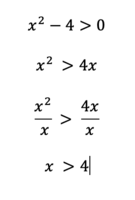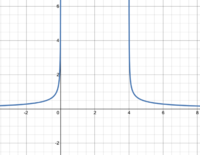# Finding the domain algebraically

#### jpanknin

##### New member
The domain of the following function is $$\displaystyle (-infinity, 0)u(4, infinity)$$:

$$\displaystyle f(x) = 1/(sqrt(x^2-4x))$$

My question is how do you find the domain algebraically? I used the following to find the $$\displaystyle (4, infinity)$$ part:

$$\displaystyle x^2 >= 4x$$

but can't figure out how to algebraically find the $$\displaystyle (-infinity, 0)$$ part. I understand that it's right, I just can find it formulaically.

#### Jomo

##### Elite Member
x2>=4x
x2- 4x>=0
Now try factoring.
Do you think say >= is correct? How about just >?

#### Dr.Peterson

##### Elite Member
The domain of the following function is (-infty, 0)cup(4, infty):

f(x) = 1/(sqrt(x^2-4x))

My question is how do you find the domain algebraically? I used the following to find the (4, infty) part:

x^2 >= 4x

but can't figure out how to algebraically find the (-infty, 0) part. I understand that it's right, I just can find it formulaically.
Jomo has reminded you of the standard way to solve a polynomial inequality. If you are not familiar with that, see for example

If you look at what I quoted from you above, you'll see that I changed your MATH codes to backquotes, which mark AsciiMath, a simplified version of LaTeX that you may find easier to work with. All I had to change in the content was "infinity" to "infty" and "u" to "cup".

#### Jomo

##### Elite Member
You have a quadratic, x^2-4x, and you want to know when it is positive. Did you graph it? Did you find the roots? Will it open upwards or downwards? How can you tell where it is positive if you know the roots and if it opens upwards or downwards?

Math is a game and you need to know the rules. Please try answering my last question above.

#### pka

##### Elite Member
The domain of the following function is $$\displaystyle (-infinity, 0)u(4, infinity)$$:
$$\displaystyle f(x) = 1/(sqrt(x^2-4x))$$
My question is how do you find the domain algebraically? I used the following to find the $$\displaystyle (4, infinity)$$ part:
My question to you: do you know how to solve inequalities in general?
Lets say, $$x^2-4x>0$$
Factor it $$x(x-4)>0$$ By inspection we see that the critical values are $$0~\&~4$$.
Those two numbers create three areas $$(-\infty,0),~(0,4),~(4,\infty)$$. Take a number from each. See which work.

•Subhotosh Khan

#### jpanknin

##### New member
Thanks, everyone. Yes, I understand inequalities and yes, I've graphed it (see below). As @Jomo pointed out it should have been > rather than >=. I calculated the (4, infty) as below on the left. So I get that part and I also see that the (-infty, 0) is valid because the x^2 will be positive for any x value and -4(x) will also be positive for any x value that is negative, meaning x^2 -4x will be positive for any value less than 0. My issue is how to find the (-infty, 0) part algebraically. I can't seem to figure out how to structure an inequality similar to the x > 4 inequality below that gives the x < 0.#### Attachments

• 12.6 KB Views: 0

#### jpanknin

##### New member
Jomo has reminded you of the standard way to solve a polynomial inequality. If you are not familiar with that, see for example

If you look at what I quoted from you above, you'll see that I changed your MATH codes to backquotes, which mark AsciiMath, a simplified version of LaTeX that you may find easier to work with. All I had to change in the content was "infinity" to "infty" and "u" to "cup".
Thanks for the backquotes tip, @Dr.Peterson. Much easier and looks much better.

#### Dr.Peterson

##### Elite Member
Thanks, everyone. Yes, I understand inequalities and yes, I've graphed it (see below). As @Jomo pointed out it should have been > rather than >=. I calculated the (4, infty) as below on the left. So I get that part and I also see that the (-infty, 0) is valid because the x^2 will be positive for any x value and -4(x) will also be positive for any x value that is negative, meaning x^2 -4x will be positive for any value less than 0. My issue is how to find the (-infty, 0) part algebraically. I can't seem to figure out how to structure an inequality similar to the x > 4 inequality below that gives the x < 0.

View attachment 24060View attachment 24058
The problem is that you can't divide both sides of an inequality by x unless you know whether it is positive or negative! Your x > 4 is not a valid conclusion.

This is why we teach the method that several of us have mentioned (#2, #5, and my links), which you claim to know but have not used.

There is an alternative method that works more like what you are trying to do, namely the use of cases:

We can say that if x > 0, then you can divide by x and get x > 4; so part of the solution is "x > 0 and x > 4", which simplifies to "x > 4".​
On the other hand, if x < 0, then when you divide by x you reverse the direction of the inequality and get x < 4. So the other part of the solution is "x < 0 and x < 4", which simplifies to "x < 0".​
Putting those together, the solution is "x > 4 or x < 0".​

I find that considerably harder than the factoring method.

#### Jomo

##### Elite Member
Thanks, everyone. Yes, I understand inequalities and yes, I've graphed it (see below). As @Jomo pointed out it should have been > rather than >=. I calculated the (4, infty) as below on the left. So I get that part and I also see that the (-infty, 0) is valid because the x^2 will be positive for any x value and -4(x) will also be positive for any x value that is negative, meaning x^2 -4x will be positive for any value less than 0. My issue is how to find the (-infty, 0) part algebraically. I can't seem to figure out how to structure an inequality similar to the x > 4 inequality below that gives the x < 0.

View attachment 24060View attachment 24058
What is this the graph of. You want to know when x^2-4x>0. So you graph y = x^2 -4x and look to see when it is greater than 0. A function is greater than 0 for each x value that has a y value >0.

The easiest way to solve x^2-4x>0 is to factor
x(x-4)>0. Note that x(x-4) is a product and that a product (of two factors) is positive when both factors are positive OR when both factors are negative.
Case 1: x>0 and x-4>0. So x> 0 AND x>4. So x>4.
Case 2: x<0 and x-4<0. So x<0 AND x<4. So x<0
Combining the answer is x<0 OR x>4.
You can write that solution in a number of different ways.